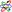## Hamilton's Equations

The equations defined by(1)(2)

whereandis the so-called Hamiltonian,are called Hamilton's equations. These equations frequently arise in problems of celestial mechanics.Another formulation related to Hamilton's equation is(3)

whereis the so-called Lagrangian.References

Morse, P. M. and Feshbach, H. Hamilton's Principle and Classical Dynamics.'' §3.2 in Methods of Theoretical Physics, Part I. New York: McGraw-Hill, pp. 280-301, 1953.